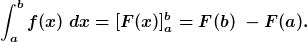# What does the antiderivative mean

## integral

1. Reverse operation for differential calculus: F (x) + C = ∫ f (x) dx with F '(x) = f (x), where F is called the antiderivative and ∫ f (x) dx is the indefinite integral, C stands for all real numbers.

2. Calculation of areas: An area between a function and the x-axis in the interval from a to b can be calculated using the determined integral: